2 Vectors

# 2.2 Coordinate Systems and Components of a Vector

### Learning Objectives

By the end of this section, you will be able to:

• Describe vectors in two and three dimensions in terms of their components, using unit vectors along the axes.
• Distinguish between the vector components of a vector and the scalar components of a vector.
• Explain how the magnitude of a vector is defined in terms of the components of a vector.
• Identify the direction angle of a vector in a plane.
• Explain the connection between polar coordinates and Cartesian coordinates in a plane.

Vectors are usually described in terms of their components in a coordinate system. Even in everyday life we naturally invoke the concept of orthogonal projections in a rectangular coordinate system. For example, if you ask someone for directions to a particular location, you will more likely be told to go 40 km east and 30 km north than 50 km in the direction $37^\circ$ north of east.

In a rectangular (Cartesian) xy-coordinate system in a plane, a point in a plane is described by a pair of coordinates (x, y). In a similar fashion, a vector $\mathbf{\overset{\to }{A}}$ in a plane is described by a pair of its vector coordinates. The x-coordinate of vector $\mathbf{\overset{\to }{A}}$ is called its x-component and the y-coordinate of vector $\mathbf{\overset{\to }{A}}$ is called its y-component. The vector x-component is a vector denoted by ${\mathbf{\overset{\to }{A}}}_{x}$. The vector y-component is a vector denoted by ${\mathbf{\overset{\to }{A}}}_{y}$. In the Cartesian system, the x and y vector components of a vector are the orthogonal projections of this vector onto the x– and y-axes, respectively. In this way, following the parallelogram rule for vector addition, each vector on a Cartesian plane can be expressed as the vector sum of its vector components:

$\mathbf{\overset{\to }{A}}={\mathbf{\overset{\to }{A}}}_{x}+{\mathbf{\overset{\to }{A}}}_{y}.$

As illustrated in Figure, vector $\mathbf{\overset{\to }{A}}$ is the diagonal of the rectangle where the x-component ${\mathbf{\overset{\to }{A}}}_{x}$ is the side parallel to the x-axis and the y-component ${\mathbf{\overset{\to }{A}}}_{y}$ is the side parallel to the y-axis. Vector component ${\mathbf{\overset{\to }{A}}}_{x}$ is orthogonal to vector component ${\mathbf{\overset{\to }{A}}}_{y}$.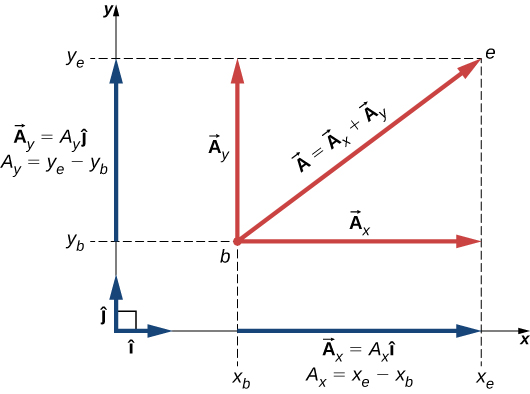Figure 2.16 Vector $\mathbf{\overset{\to }{A}}$ in a plane in the Cartesian coordinate system is the vector sum of its vector x- and y-components. The x-vector component ${\mathbf{\overset{\to }{A}}}_{x}$ is the orthogonal projection of vector $\mathbf{\overset{\to }{A}}$ onto the x-axis. The y-vector component ${\mathbf{\overset{\to }{A}}}_{y}$ is the orthogonal projection of vector $\mathbf{\overset{\to }{A}}$ onto the y-axis. The numbers ${A}_{x}$ and ${A}_{y}$ that multiply the unit vectors are the scalar components of the vector.

It is customary to denote the positive direction on the x-axis by the unit vector $\mathbf{\hat{i}}$ and the positive direction on the y-axis by the unit vector $\mathbf{\hat{j}}$. Unit vectors of the axes, $\mathbf{\hat{i}}$ and $\mathbf{\hat{j}}$, define two orthogonal directions in the plane. As shown in Figure, the x– and y– components of a vector can now be written in terms of the unit vectors of the axes:

$\left\{\begin{array}{c}{\mathbf{\overset{\to }{A}}}_{x}={A}_{x}\mathbf{\hat{i}}\\ {\mathbf{\overset{\to }{A}}}_{y}={A}_{y}\mathbf{\hat{j}}.\end{array}\right.$

The vectors ${\mathbf{\overset{\to }{A}}}_{x}$ and ${\mathbf{\overset{\to }{A}}}_{y}$ defined by Figure are the vector components of vector $\mathbf{\overset{\to }{A}}$. The numbers ${A}_{x}$ and ${A}_{y}$ that define the vector components in Figure are the scalar components of vector $\mathbf{\overset{\to }{A}}$. Combining Figure with Figure, we obtain the component form of a vector:

$\mathbf{\overset{\to }{A}}={A}_{x}\mathbf{\hat{i}}+{A}_{y}\mathbf{\hat{j}}.$

If we know the coordinates $b({x}_{b},{y}_{b})$ of the origin point of a vector (where b stands for “beginning”) and the coordinates $e({x}_{e},{y}_{e})$ of the end point of a vector (where e stands for “end”), we can obtain the scalar components of a vector simply by subtracting the origin point coordinates from the end point coordinates:

$\left\{\begin{array}{c}{A}_{x}={x}_{e}-{x}_{b}\\ {A}_{y}={y}_{e}-{y}_{b}.\end{array}\right.$

### Example

#### Displacement of a Mouse Pointer

A mouse pointer on the display monitor of a computer at its initial position is at point (6.0 cm, 1.6 cm) with respect to the lower left-side corner. If you move the pointer to an icon located at point (2.0 cm, 4.5 cm), what is the displacement vector of the pointer?

#### Strategy

The origin of the xy-coordinate system is the lower left-side corner of the computer monitor. Therefore, the unit vector $\mathbf{\hat{i}}$ on the x-axis points horizontally to the right and the unit vector $\mathbf{\hat{j}}$ on the y-axis points vertically upward. The origin of the displacement vector is located at point b(6.0, 1.6) and the end of the displacement vector is located at point e(2.0, 4.5). Substitute the coordinates of these points into Figure to find the scalar components ${D}_{x}$ and ${D}_{y}$ of the displacement vector $\mathbf{\overset{\to }{D}}$. Finally, substitute the coordinates into Figure to write the displacement vector in the vector component form.

#### Solution

We identify ${x}_{b}=6.0$, ${x}_{e}=2.0$, ${y}_{b}=1.6$, and ${y}_{e}=4.5$, where the physical unit is 1 cm. The scalar x- and y-components of the displacement vector are $\begin{array}{cc}\hfill {D}_{x}& ={x}_{e}-{x}_{b}=(2.0-6.0)\text{cm}=-4.0\,\text{cm},\hfill \\ \hfill {D}_{y}& ={y}_{e}-{y}_{b}=(4.5-1.6)\text{cm}=+2.9\,\text{cm}.\hfill \end{array}$

The vector component form of the displacement vector is

$\mathbf{\overset{\to }{D}}={D}_{x}\mathbf{\hat{i}}+{D}_{y}\mathbf{\hat{j}}=(-4.0\,\text{cm})\mathbf{\hat{i}}+(2.9\,\text{cm})\mathbf{\hat{j}}=(-4.0\mathbf{\hat{i}}+2.9\mathbf{\hat{j}})\text{cm}.$This solution is shown in (Figure 2.17).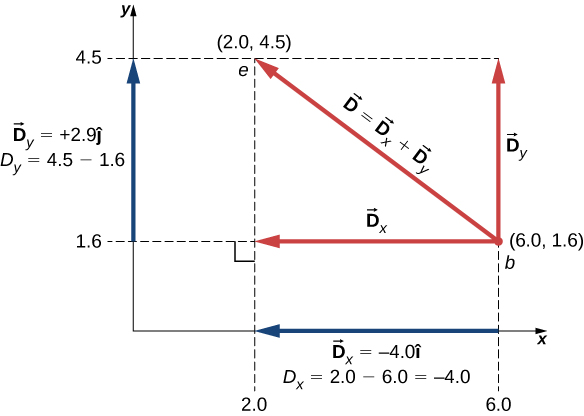Figure 2.17 The graph of the displacement vector. The vector points from the origin point at b to the end point at e.

#### Significance

Notice that the physical unit—here, 1 cm—can be placed either with each component immediately before the unit vector or globally for both components, as in Figure. Often, the latter way is more convenient because it is simpler.

The vector x-component ${\mathbf{\overset{\to }{D}}}_{x}=-4.0\mathbf{\hat{i}}=4.0(\text{−}\mathbf{\hat{i}})$ of the displacement vector has the magnitude $|{\mathbf{\overset{\to }{D}}}_{x}|=|-4.0||\mathbf{\hat{i}}|=4.0$ because the magnitude of the unit vector is $|\mathbf{\hat{i}}|=1$. Notice, too, that the direction of the x-component is $\text{−}\mathbf{\hat{i}}$, which is antiparallel to the direction of the +x-axis; hence, the x-component vector ${\mathbf{\overset{\to }{D}}}_{x}$ points to the left, as shown in Figure. The scalar x-component of vector $\mathbf{\overset{\to }{D}}$ is ${D}_{x}=-4.0$.

Similarly, the vector y-component ${\mathbf{\overset{\to }{D}}}_{y}=+2.9\mathbf{\hat{j}}$ of the displacement vector has magnitude $|{\mathbf{\overset{\to }{D}}}_{y}|=|2.9||\mathbf{\hat{j}}|=\,2.9$ because the magnitude of the unit vector is $|\mathbf{\hat{j}}|=1$. The direction of the y-component is $+\mathbf{\hat{j}}$, which is parallel to the direction of the +y-axis. Therefore, the y-component vector ${\mathbf{\overset{\to }{D}}}_{y}$ points up, as seen in Figure. The scalar y-component of vector $\mathbf{\overset{\to }{D}}$ is ${D}_{y}=+2.9$. The displacement vector $\mathbf{\overset{\to }{D}}$ is the resultant of its two vector components.

The vector component form of the displacement vector Figure tells us that the mouse pointer has been moved on the monitor 4.0 cm to the left and 2.9 cm upward from its initial position.

A blue fly lands on a sheet of graph paper at a point located 10.0 cm to the right of its left edge and 8.0 cm above its bottom edge and walks slowly to a point located 5.0 cm from the left edge and 5.0 cm from the bottom edge. Choose the rectangular coordinate system with the origin at the lower left-side corner of the paper and find the displacement vector of the fly. Illustrate your solution by graphing.

Show Solution

$\mathbf{\overset{\to }{D}}=(-5.0\mathbf{\hat{i}}-3.0\mathbf{\hat{j}})\text{cm}$; the fly moved 5.0 cm to the left and 3.0 cm down from its landing site.

When we know the scalar components ${A}_{x}$ and ${A}_{y}$ of a vector $\mathbf{\overset{\to }{A}}$, we can find its magnitude A and its direction angle ${\theta }_{A}$. The direction angle—or direction, for short—is the angle the vector forms with the positive direction on the x-axis. The angle ${\theta }_{A}$ is measured in the counterclockwise direction from the +x-axis to the vector (Figure). Because the lengths A, ${A}_{x}$, and ${A}_{y}$ form a right triangle, they are related by the Pythagorean theorem:

${A}^{2}={A}_{x}^{2}+{A}_{y}^{2}\enspace\Leftrightarrow\enspace{A}=\sqrt{{A}_{x}^{2}+{A}_{y}^{2}}.$

This equation works even if the scalar components of a vector are negative. The direction angle ${\theta }_{A}$ of a vector is defined via the tangent function of angle ${\theta }_{A}$ in the triangle shown in Figure:

$\text{tan}\,{\theta }_{A}=\frac{{A}_{y}}{{A}_{x}}\enspace\Rightarrow \enspace{\theta }_{A}={\text{tan}}^{-1}(\frac{{A}_{y}}{{A}_{x}}).$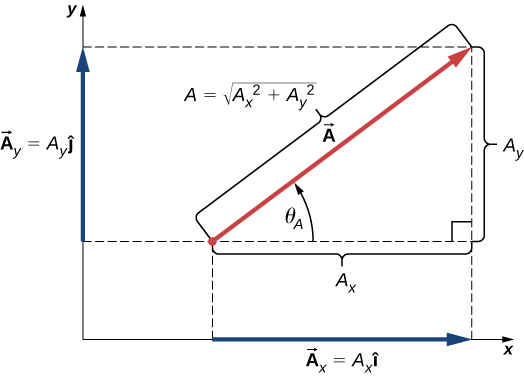Figure 2.18 For vector $\mathbf{\overset{\to }{A}}$, its magnitude A and its direction angle ${\theta }_{A}$ are related to the magnitudes of its scalar components because A, ${A}_{x}$, and ${A}_{y}$ form a right triangle.

When the vector lies either in the first quadrant or in the fourth quadrant, where component ${A}_{x}$ is positive (Figure), the angle $\theta$ in Figure is identical to the direction angle ${\theta }_{A}$. For vectors in the fourth quadrant, angle $\theta$ is negative, which means that for these vectors, direction angle ${\theta }_{A}$ is measured clockwise from the positive x-axis. Similarly, for vectors in the second quadrant, angle $\theta$ is negative. When the vector lies in either the second or third quadrant, where component ${A}_{x}$ is negative, the direction angle is ${\theta }_{A}=\theta +180^\circ$ (Figure).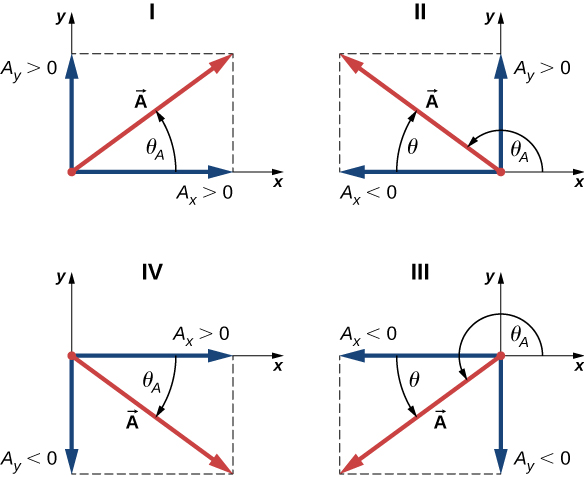Figure 2.19 Scalar components of a vector may be positive or negative. Vectors in the first quadrant (I) have both scalar components positive and vectors in the third quadrant have both scalar components negative. For vectors in quadrants II and III, the direction angle of a vector is ${\theta }_{A}=\theta +180^\circ$.

### Example

#### Magnitude and Direction of the Displacement Vector

You move a mouse pointer on the display monitor from its initial position at point (6.0 cm, 1.6 cm) to an icon located at point (2.0 cm, 4.5 cm). What are the magnitude and direction of the displacement vector of the pointer?

#### Strategy

In Figure, we found the displacement vector $\mathbf{\overset{\to }{D}}$ of the mouse pointer (see Figure). We identify its scalar components ${D}_{x}=-4.0\,\text{cm}$ and ${D}_{y}=+2.9\,\text{cm}$ and substitute into Figure and Figure to find the magnitude D and direction ${\theta }_{D}$, respectively.

#### Solution

The magnitude of vector $\mathbf{\overset{\to }{D}}$ is $D=\sqrt{{D}_{x}^{2}+{D}_{y}^{2}}=\sqrt{{(-4.0\,\text{cm})}^{2}+{(2.9\,\text{cm})}^{2}}=\sqrt{{(4.0)}^{2}+{(2.9)}^{2}}\,\text{cm}=4.9\,\text{cm}.$ The direction angle is $\text{tan}\,\theta =\frac{{D}_{y}}{{D}_{x}}=\frac{+2.9\,\text{cm}}{-4.0\,\text{cm}}=-0.725\enspace\Rightarrow \enspace\theta ={\text{tan}}^{-1}(-0.725)=-35.9^\circ.$ Vector $\mathbf{\overset{\to }{D}}$ lies in the second quadrant, so its direction angle is ${\theta }_{D}=\theta +180^\circ=-35.9^\circ+180^\circ=144.1^\circ.$

If the displacement vector of a blue fly walking on a sheet of graph paper is $\mathbf{\overset{\to }{D}}=(-5.00\mathbf{\hat{i}}-3.00\mathbf{\hat{j}})\text{cm}$, find its magnitude and direction.

Show Solution

5.83 cm, $211^\circ$

In many applications, the magnitudes and directions of vector quantities are known and we need to find the resultant of many vectors. For example, imagine 400 cars moving on the Golden Gate Bridge in San Francisco in a strong wind. Each car gives the bridge a different push in various directions and we would like to know how big the resultant push can possibly be. We have already gained some experience with the geometric construction of vector sums, so we know the task of finding the resultant by drawing the vectors and measuring their lengths and angles may become intractable pretty quickly, leading to huge errors. Worries like this do not appear when we use analytical methods. The very first step in an analytical approach is to find vector components when the direction and magnitude of a vector are known.

Let us return to the right triangle in Figure. The quotient of the adjacent side ${A}_{x}$ to the hypotenuse A is the cosine function of direction angle ${\theta }_{A}$, ${A}_{x}\text{/}A=\text{cos}\,{\theta }_{A}$, and the quotient of the opposite side ${A}_{y}$ to the hypotenuse A is the sine function of ${\theta }_{A}$, ${A}_{y}\text{/}A=\text{sin}\,{\theta }_{A}$. When magnitude A and direction ${\theta }_{A}$ are known, we can solve these relations for the scalar components:

$\left\{\begin{array}{c}{A}_{x}=A\,\text{cos}\,{\theta }_{A}\\ {A}_{y}=A\,\text{sin}\,{\theta }_{A}\end{array}\right. .$

When calculating vector components with Figure, care must be taken with the angle. The direction angle ${\theta }_{A}$ of a vector is the angle measured counterclockwise from the positive direction on the x-axis to the vector. The clockwise measurement gives a negative angle.

### Example

#### Components of Displacement Vectors

A rescue party for a missing child follows a search dog named Trooper. Trooper wanders a lot and makes many trial sniffs along many different paths. Trooper eventually finds the child and the story has a happy ending, but his displacements on various legs seem to be truly convoluted. On one of the legs he walks 200.0 m southeast, then he runs north some 300.0 m. On the third leg, he examines the scents carefully for 50.0 m in the direction $30^\circ$ west of north. On the fourth leg, Trooper goes directly south for 80.0 m, picks up a fresh scent and turns $23^\circ$ west of south for 150.0 m. Find the scalar components of Trooper’s displacement vectors and his displacement vectors in vector component form for each leg.

#### Strategy

Let’s adopt a rectangular coordinate system with the positive x-axis in the direction of geographic east, with the positive y-direction pointed to geographic north. Explicitly, the unit vector $\mathbf{\hat{i}}$ of the x-axis points east and the unit vector $\mathbf{\hat{j}}$ of the y-axis points north. Trooper makes five legs, so there are five displacement vectors. We start by identifying their magnitudes and direction angles, then we use Figure to find the scalar components of the displacements and Figure for the displacement vectors.

#### Solution

On the first leg, the displacement magnitude is ${L}_{1}=200.0\,\text{m}$ and the direction is southeast. For direction angle ${\theta }_{1}$ we can take either $45^\circ$ measured clockwise from the east direction or $45^\circ+270^\circ$ measured counterclockwise from the east direction. With the first choice, ${\theta }_{1}=-45^\circ$. With the second choice, ${\theta }_{1}=+315^\circ$. We can use either one of these two angles. The components are

$\begin{array}{l}{L}_{1x}={L}_{1}\,\text{cos}\,{\theta }_{1}=(200.0\,\text{m})\,\text{cos}\,315^\circ=141.4\,\text{m,}\\ {L}_{1y}={L}_{1}\,\text{sin}\,{\theta }_{1}=(200.0\,\text{m})\,\text{sin}\,315^\circ=-141.4\,\text{m}.\end{array}$

The displacement vector of the first leg is

${\mathbf{\overset{\to }{L}}}_{1}={L}_{1x}\mathbf{\hat{i}}+{L}_{1y}\mathbf{\hat{j}}=(141.4\mathbf{\hat{i}}-141.4\mathbf{\hat{j}})\,\text{m}.$

On the second leg of Trooper’s wanderings, the magnitude of the displacement is ${L}_{2}=300.0\,\text{m}$ and the direction is north. The direction angle is ${\theta }_{2}=+90^\circ$. We obtain the following results:

$\begin{array}{ccc}\hfill {L}_{2x}& =\hfill & {L}_{2}\,\text{cos}\,{\theta }_{2}=(300.0\,\text{m})\,\text{cos}\,90^\circ=0.0\,,\hfill \\ \hfill {L}_{2y}& =\hfill & {L}_{2}\,\text{sin}\,{\theta }_{2}=(300.0\,\text{m})\,\text{sin}\,90^\circ=300.0\,\text{m,}\hfill \\ \hfill {\mathbf{\overset{\to }{L}}}_{2}& =\hfill & {L}_{2x}\mathbf{\hat{i}}+{L}_{2y}\mathbf{\hat{j}}=(300.0\,\text{m})\mathbf{\hat{j}}.\hfill \end{array}$

On the third leg, the displacement magnitude is ${L}_{3}=50.0\,\text{m}$ and the direction is $30^\circ$ west of north. The direction angle measured counterclockwise from the eastern direction is ${\theta }_{3}=30^\circ+90^\circ=+120^\circ$. This gives the following answers:

$\begin{array}{ccc}\hfill {L}_{3x}& =\hfill & {L}_{3}\,\text{cos}\,{\theta }_{3}=(50.0\,\text{m})\,\text{cos}\,120^\circ=-25.0\,\text{m,}\hfill \\ \hfill {L}_{3y}& =\hfill & {L}_{3}\,\text{sin}\,{\theta }_{3}=(50.0\,\text{m})\,\text{sin}\,120^\circ=+43.3\,\text{m,}\hfill \\ \hfill {\mathbf{\overset{\to }{L}}}_{3}& =\hfill & {L}_{3x}\mathbf{\hat{i}}+{L}_{3y}\mathbf{\hat{j}}=(-25.0\mathbf{\hat{i}}+43.3\mathbf{\hat{j}})\text{m}.\hfill \end{array}$

On the fourth leg of the excursion, the displacement magnitude is ${L}_{4}=80.0\,\text{m}$ and the direction is south. The direction angle can be taken as either ${\theta }_{4}=-90^\circ$ or ${\theta }_{4}=+270^\circ$. We obtain

$\begin{array}{ccc}\hfill {L}_{4x}& =\hfill & {L}_{4}\,\text{cos}\,{\theta }_{4}=(80.0\,\text{m})\,\text{cos}\,(-90^\circ)=0\,,\hfill \\ \hfill {L}_{4y}& =\hfill & {L}_{4}\,\text{sin}\,{\theta }_{4}=(80.0\,\text{m})\,\text{sin}\,(-90^\circ)=-80.0\,\text{m,}\hfill \\ \hfill {\mathbf{\overset{\to }{L}}}_{4}& =\hfill & {L}_{4x}\mathbf{\hat{i}}+{L}_{4y}\mathbf{\hat{j}}=(-80.0\,\text{m})\mathbf{\hat{j}}.\hfill \end{array}$

On the last leg, the magnitude is ${L}_{5}=150.0\,\text{m}$ and the angle is ${\theta }_{5}=-23^\circ+270^\circ=+247^\circ$ $(23^\circ$ west of south), which gives $\begin{array}{ccc}\hfill {L}_{5x}& =\hfill & {L}_{5}\,\text{cos}\,{\theta }_{5}=(150.0\,\text{m})\,\text{cos}\,247^\circ=-58.6\,\text{m,}\hfill \\ \hfill {L}_{5y}& =\hfill & {L}_{5}\,\text{sin}\,{\theta }_{5}=(150.0\,\text{m})\,\text{sin}\,247^\circ=-138.1\,\text{m,}\hfill \\ \hfill {\mathbf{\overset{\to }{L}}}_{5}& =\hfill & {L}_{5x}\mathbf{\hat{i}}+{L}_{5y}\mathbf{\hat{j}}=(-58.6\mathbf{\hat{i}}-138.1\mathbf{\hat{j}})\text{m}.\hfill \end{array}$

If Trooper runs 20 m west before taking a rest, what is his displacement vector?

Show Solution

$\mathbf{\overset{\to }{D}}=(-20\,\text{m})\mathbf{\hat{j}}$

### Polar Coordinates

To describe locations of points or vectors in a plane, we need two orthogonal directions. In the Cartesian coordinate system these directions are given by unit vectors $\mathbf{\hat{i}}$ and $\mathbf{\hat{j}}$ along the x-axis and the y-axis, respectively. The Cartesian coordinate system is very convenient to use in describing displacements and velocities of objects and the forces acting on them. However, it becomes cumbersome when we need to describe the rotation of objects. When describing rotation, we usually work in the polar coordinate system.

In the polar coordinate system, the location of point P in a plane is given by two polar coordinates (Figure). The first polar coordinate is the radial coordinate r, which is the distance of point P from the origin. The second polar coordinate is an angle $\phi$ that the radial vector makes with some chosen direction, usually the positive x-direction. In polar coordinates, angles are measured in radians, or rads. The radial vector is attached at the origin and points away from the origin to point P. This radial direction is described by a unit radial vector $\mathbf{\hat{r}}$. The second unit vector $\mathbf{\hat{t}}$ is a vector orthogonal to the radial direction $\mathbf{\hat{r}}$. The positive $+\mathbf{\hat{t}}$ direction indicates how the angle $\phi$ changes in the counterclockwise direction. In this way, a point P that has coordinates (x, y) in the rectangular system can be described equivalently in the polar coordinate system by the two polar coordinates $(r,\phi )$. Figure is valid for any vector, so we can use it to express the x– and y-coordinates of vector $\mathbf{\overset{\to }{r}}$. In this way, we obtain the connection between the polar coordinates and rectangular coordinates of point P:

$\left\{\begin{array}{c}x=r\,\text{cos}\,\phi \\ y=r\,\text{sin}\,\phi \end{array}\right. .$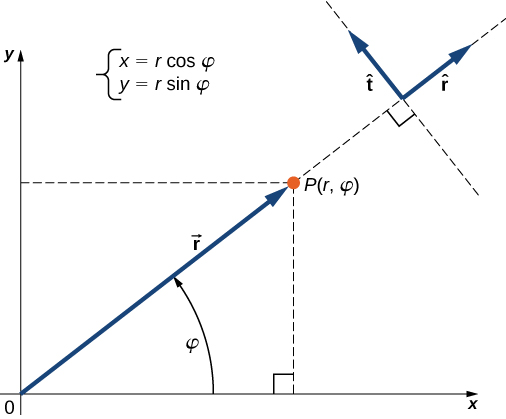Figure 2.20 Using polar coordinates, the unit vector $\mathbf{\hat{r}}$ defines the positive direction along the radius r (radial direction) and, orthogonal to it, the unit vector $\mathbf{\hat{t}}$ defines the positive direction of rotation by the angle $\phi$.

### Example

#### Polar Coordinates

A treasure hunter finds one silver coin at a location 20.0 m away from a dry well in the direction $20^\circ$ north of east and finds one gold coin at a location 10.0 m away from the well in the direction $20^\circ$ north of west. What are the polar and rectangular coordinates of these findings with respect to the well?

#### Strategy

The well marks the origin of the coordinate system and east is the +x-direction. We identify radial distances from the locations to the origin, which are ${r}_{S}=20.0\,\text{m}$ (for the silver coin) and ${r}_{G}=10.0\,\text{m}$ (for the gold coin). To find the angular coordinates, we convert $20^\circ$ to radians: $20^\circ=\pi 20\text{/}180=\pi \text{/}9$. We use Figure to find the x– and y-coordinates of the coins.

#### Solution

The angular coordinate of the silver coin is ${\phi }_{S}=\pi \text{/}9$, whereas the angular coordinate of the gold coin is ${\phi }_{G}=\pi -\pi \text{/}9=8\pi \text{/}9$. Hence, the polar coordinates of the silver coin are $({r}_{S},{\phi }_{S})=(20.0\,\text{m},\pi \text{/}9)$ and those of the gold coin are $({r}_{G},{\phi }_{G})=(10.0\,\text{m},8\pi \text{/}9)$. We substitute these coordinates into (Figure) to obtain rectangular coordinates. For the gold coin, the coordinates are

$\left\{\begin{array}{l}{x}_{G}={r}_{G}\,\text{cos}\,{\phi }_{G}=(10.0\,\text{m})\,\text{cos}\,8\pi \text{/}9=-9.4\,\text{m}\\ {y}_{G}={r}_{G}\,\text{sin}\,{\phi }_{G}=(10.0\,\text{m})\,\text{sin}\,8\pi \text{/}9=3.4\,\text{m}\end{array}\right. \enspace\Rightarrow \enspace({x}_{G},{y}_{G})=(-9.4\,\text{m},3.4\,\text{m}).$

For the silver coin, the coordinates are

$\left\{\begin{array}{l}{x}_{S}={r}_{S}\,\text{cos}\,{\phi }_{S}=(20.0\,\text{m})\,\text{cos}\,\pi \text{/}9=18.9\,\text{m}\\ {y}_{S}={r}_{S}\,\text{sin}\,{\phi }_{S}=(20.0\,\text{m})\,\text{sin}\,\pi \text{/}9=6.8\,\text{m}\end{array}\right.\enspace\Rightarrow \enspace({x}_{S},{y}_{S})=(18.9\,\text{m},6.8\,\text{m}).$

### Vectors in Three Dimensions

To specify the location of a point in space, we need three coordinates (x, y, z), where coordinates x and y specify locations in a plane, and coordinate z gives a vertical position above or below the plane. Three-dimensional space has three orthogonal directions, so we need not two but three unit vectors to define a three-dimensional coordinate system. In the Cartesian coordinate system, the first two unit vectors are the unit vector of the x-axis $\mathbf{\hat{i}}$ and the unit vector of the y-axis $\mathbf{\hat{j}}$. The third unit vector $\mathbf{\hat{k}}$ is the direction of the z-axis (Figure). The order in which the axes are labeled, which is the order in which the three unit vectors appear, is important because it defines the orientation of the coordinate system. The order xyz, which is equivalent to the order $\mathbf{\hat{i}}$ – $\mathbf{\hat{j}}$ – $\mathbf{\hat{k}}$, defines the standard right-handed coordinate system (positive orientation).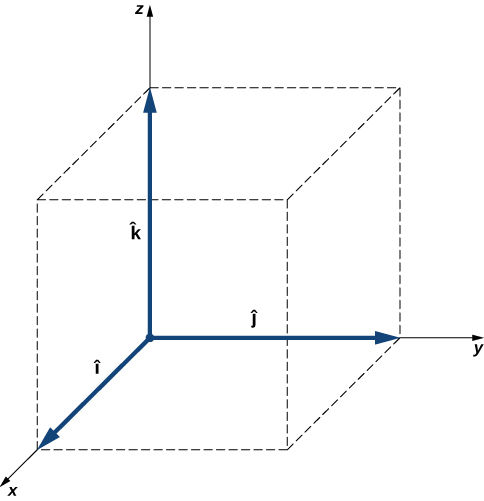Figure 2.21 Three unit vectors define a Cartesian system in three-dimensional space. The order in which these unit vectors appear defines the orientation of the coordinate system. The order shown here defines the right-handed orientation.

In three-dimensional space, vector $\mathbf{\overset{\to }{A}}$ has three vector components: the x-component ${\mathbf{\overset{\to }{A}}}_{x}={A}_{x}\mathbf{\hat{i}}$, which is the part of vector $\mathbf{\overset{\to }{A}}$ along the x-axis; the y-component ${\mathbf{\overset{\to }{A}}}_{y}={A}_{y}\mathbf{\hat{j}}$, which is the part of $\mathbf{\overset{\to }{A}}$ along the y-axis; and the z-component ${\mathbf{\overset{\to }{A}}}_{z}={A}_{z}\mathbf{\hat{k}}$, which is the part of the vector along the z-axis. A vector in three-dimensional space is the vector sum of its three vector components (Figure):

$\mathbf{\overset{\to }{A}}={A}_{x}\mathbf{\hat{i}}+{A}_{y}\mathbf{\hat{j}}+{A}_{z}\mathbf{\hat{k}}.$

If we know the coordinates of its origin $b({x}_{b},{y}_{b},{z}_{b})$ and of its end $e({x}_{e},{y}_{e},{z}_{e})$, its scalar components are obtained by taking their differences: ${A}_{x}$ and ${A}_{y}$ are given by Figure and the z-component is given by

${A}_{z}={z}_{e}-{z}_{b}.$

Magnitude A is obtained by generalizing Figure to three dimensions:

$A=\sqrt{{A}_{x}^{2}+{A}_{y}^{2}+{A}_{z}^{2}}.$

This expression for the vector magnitude comes from applying the Pythagorean theorem twice. As seen in Figure, the diagonal in the xy-plane has length $\sqrt{{A}_{x}^{2}+{A}_{y}^{2}}$ and its square adds to the square ${A}_{z}^{2}$ to give ${A}^{2}$. Note that when the z-component is zero, the vector lies entirely in the xy-plane and its description is reduced to two dimensions.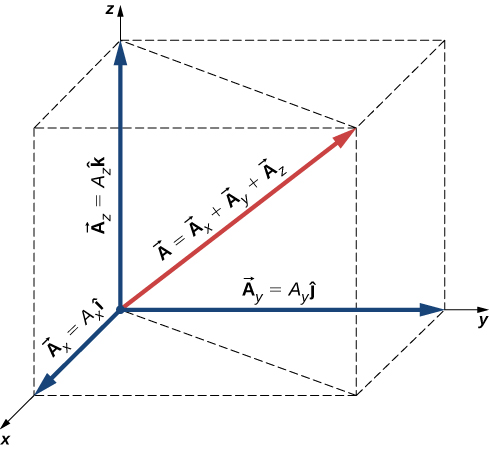Figure 2.22 A vector in three-dimensional space is the vector sum of its three vector components.

### Example

#### Takeoff of a Drone

During a takeoff of IAI Heron (Figure), its position with respect to a control tower is 100 m above the ground, 300 m to the east, and 200 m to the north. One minute later, its position is 250 m above the ground, 1200 m to the east, and 2100 m to the north. What is the drone’s displacement vector with respect to the control tower? What is the magnitude of its displacement vector?

#### Strategy

We take the origin of the Cartesian coordinate system as the control tower. The direction of the +x-axis is given by unit vector $\mathbf{\hat{i}}$ to the east, the direction of the +y-axis is given by unit vector $\mathbf{\hat{j}}$ to the north, and the direction of the +z-axis is given by unit vector $\mathbf{\hat{k}}$, which points up from the ground. The drone’s first position is the origin (or, equivalently, the beginning) of the displacement vector and its second position is the end of the displacement vector.

#### Solution

We identify b(300.0 m, 200.0 m, 100.0 m) and e(480.0 m, 370.0 m, 250.0m), and use (Figure) and (Figure) to find the scalar components of the drone’s displacement vector:

$\left\{\begin{array}{l}{D}_{x}={x}_{e}-{x}_{b}=1200.0\,\text{m}-300.0\,\text{m}=900.0\,\text{m},\\ {D}_{y}={y}_{e}-{y}_{b}=2100.0\,\text{m}-200.0\,\text{m}=1900.0\,\text{m,}\\ {D}_{z}={z}_{e}-{z}_{b}=250.0\,\text{m}-100.0\,\text{m}=150.0\,\text{m}.\end{array}\right.$

We substitute these components into (Figure) to find the displacement vector:

$\mathbf{\overset{\to }{D}}={D}_{x}\mathbf{\hat{i}}+{D}_{y}\mathbf{\hat{j}}+{D}_{z}\mathbf{\hat{k}}=900.0\,\text{m}\mathbf{\hat{i}}+1900.0\,\text{m}\mathbf{\hat{j}}+150.0\,\text{m}\mathbf{\hat{k}}=(0.90\mathbf{\hat{i}}+1.90\mathbf{\hat{j}}+0.15\mathbf{\hat{k}})\,\text{km}.$

We substitute into (Figure) to find the magnitude of the displacement: $D=\sqrt{{D}_{x}^{2}+{D}_{y}^{2}+{D}_{z}^{2}}=\sqrt{{(0.90\,\text{km})}^{2}+{(1.90\,\text{km})}^{2}+{(0.15\,\text{km})}^{2}}=4.44\,\text{km}.$

If the average velocity vector of the drone in the displacement in Figure is $\mathbf{\overset{\to }{u}}=(15.0\mathbf{\hat{i}}+31.7\mathbf{\hat{j}}+2.5\mathbf{\hat{k}})\text{m}\text{/}\text{s}$, what is the magnitude of the drone’s velocity vector?

Show Solution

35.1 m/s = 126.4 km/h

### Summary

• Vectors are described in terms of their components in a coordinate system. In two dimensions (in a plane), vectors have two components. In three dimensions (in space), vectors have three components.
• A vector component of a vector is its part in an axis direction. The vector component is the product of the unit vector of an axis with its scalar component along this axis. A vector is the resultant of its vector components.
• Scalar components of a vector are differences of coordinates, where coordinates of the origin are subtracted from end point coordinates of a vector. In a rectangular system, the magnitude of a vector is the square root of the sum of the squares of its components.
• In a plane, the direction of a vector is given by an angle the vector has with the positive x-axis. This direction angle is measured counterclockwise. The scalar x-component of a vector can be expressed as the product of its magnitude with the cosine of its direction angle, and the scalar y-component can be expressed as the product of its magnitude with the sine of its direction angle.
• In a plane, there are two equivalent coordinate systems. The Cartesian coordinate system is defined by unit vectors $\mathbf{\hat{i}}$ and $\mathbf{\hat{j}}$ along the x-axis and the y-axis, respectively. The polar coordinate system is defined by the radial unit vector $\mathbf{\hat{r}}$, which gives the direction from the origin, and a unit vector $\mathbf{\hat{t}}$, which is perpendicular (orthogonal) to the radial direction.

### Conceptual Questions

Give an example of a nonzero vector that has a component of zero.

Show Solution

a unit vector of the x-axis

Explain why a vector cannot have a component greater than its own magnitude.

If two vectors are equal, what can you say about their components?

Show Solution

They are equal.

If vectors $\mathbf{\overset{\to }{A}}$ and $\mathbf{\overset{\to }{B}}$ are orthogonal, what is the component of $\mathbf{\overset{\to }{B}}$ along the direction of $\mathbf{\overset{\to }{A}}$? What is the component of $\mathbf{\overset{\to }{A}}$ along the direction of $\mathbf{\overset{\to }{B}}$?

If one of the two components of a vector is not zero, can the magnitude of the other vector component of this vector be zero?

Show Solution

yes

If two vectors have the same magnitude, do their components have to be the same?

### Problems

Assuming the +x-axis is horizontal and points to the right, resolve the vectors given in the following figure to their scalar components and express them in vector component form.

Show Solution

a. $\mathbf{\overset{\to }{A}}=+8.66\mathbf{\hat{i}}+5.00\mathbf{\hat{j}}$, b. $\mathbf{\overset{\to }{B}}=+30.09\mathbf{\hat{i}}+39.93\mathbf{\hat{j}}$, c. $\mathbf{\overset{\to }{C}}=+6.00\mathbf{\hat{i}}-10.39\mathbf{\hat{j}}$, d. $\mathbf{\overset{\to }{D}}=-15.97\mathbf{\hat{i}}+12.04\mathbf{\hat{j}}$, f. $\mathbf{\overset{\to }{F}}=-17.32\mathbf{\hat{i}}-10.00\mathbf{\hat{j}}$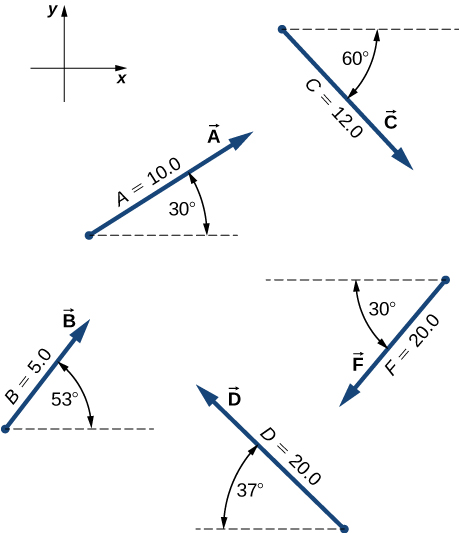Suppose you walk 18.0 m straight west and then 25.0 m straight north. How far are you from your starting point? What is your displacement vector? What is the direction of your displacement? Assume the +x-axis is horizontal to the right.

You drive 7.50 km in a straight line in a direction $15^\circ$ east of north. (a) Find the distances you would have to drive straight east and then straight north to arrive at the same point. (b) Show that you still arrive at the same point if the east and north legs are reversed in order. Assume the +x-axis is to the east.

Show Solution

a. 1.94 km, 7.24 km; b. proof

A sledge is being pulled by two horses on a flat terrain. The net force on the sledge can be expressed in the Cartesian coordinate system as vector $\mathbf{\overset{\to }{F}}=(-2980.0\mathbf{\hat{i}}+8200.0\mathbf{\hat{j}})\text{N}$, where $\mathbf{\hat{i}}$ and $\mathbf{\hat{j}}$ denote directions to the east and north, respectively. Find the magnitude and direction of the pull.

A trapper walks a 5.0-km straight-line distance from her cabin to the lake, as shown in the following figure. Determine the east and north components of her displacement vector. How many more kilometers would she have to walk if she walked along the component displacements? What is her displacement vector?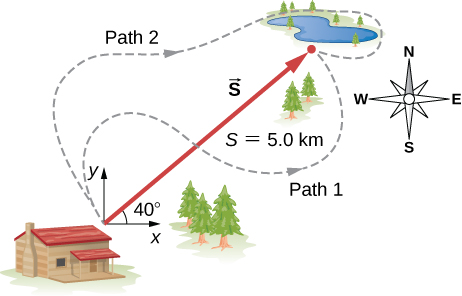3.8 km east, 3.2 km north, 2.0 km, $\mathbf{\overset{\to }{D}}=(3.8\mathbf{\hat{i}}+3.2\mathbf{\hat{j}})\text{km}$

The polar coordinates of a point are $4\pi \text{/}3$ and 5.50 m. What are its Cartesian coordinates?

Two points in a plane have polar coordinates ${P}_{1}(2.500\,\text{m},\pi \text{/}6)$ and ${P}_{2}(3.800\,\text{m},2\pi \text{/}3)$. Determine their Cartesian coordinates and the distance between them in the Cartesian coordinate system. Round the distance to a nearest centimeter.

Show Solution

${P}_{1}(2.165\,\text{m},1.250\,\text{m})$, ${P}_{2}(-1.900\,\text{m},3.290\,\text{m})$, 5.27 m

A chameleon is resting quietly on a lanai screen, waiting for an insect to come by. Assume the origin of a Cartesian coordinate system at the lower left-hand corner of the screen and the horizontal direction to the right as the +x-direction. If its coordinates are (2.000 m, 1.000 m), (a) how far is it from the corner of the screen? (b) What is its location in polar coordinates?

Two points in the Cartesian plane are A(2.00 m, −4.00 m) and B(−3.00 m, 3.00 m). Find the distance between them and their polar coordinates.

Show Solution

8.60 m, $A(2\sqrt{5}\,\text{m},0.647\pi )$, $B(3\sqrt{2}\,\text{m},0.75\pi )$

A fly enters through an open window and zooms around the room. In a Cartesian coordinate system with three axes along three edges of the room, the fly changes its position from point b(4.0 m, 1.5 m, 2.5 m) to point e(1.0 m, 4.5 m, 0.5 m). Find the scalar components of the fly’s displacement vector and express its displacement vector in vector component form. What is its magnitude?

### Glossary

component form of a vector
a vector written as the vector sum of its components in terms of unit vectors
direction angle
in a plane, an angle between the positive direction of the x-axis and the vector, measured counterclockwise from the axis to the vector
polar coordinate system
an orthogonal coordinate system where location in a plane is given by polar coordinates
polar coordinates
a radial coordinate and an angle